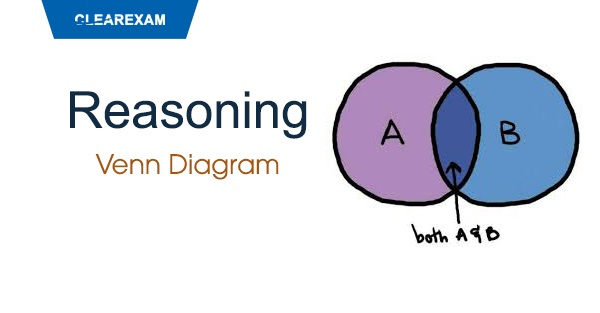## [LATEST]\$type=sticky\$show=home\$rm=0\$va=0\$count=4\$va=0

Reasoning is an important subject in CTET,CLAT & Other UG Entrance Exams. In any of entrance exam, Venn Diagram carries a good weightage of questions. With focused practice good marks can be fetched from this section. These questions are very important in achieving your success in all entrance exams.

DIRECTION(1-10): EACH OF THE FOLLOWING QUESTIONS, CONTAIN THREE ITEMS.USING THE RELATIONSHIP BETWEEN THESE ITEMS MATCH EACH QUESTION WITH THE MOST SUITABLE DIAGRAM.

(A)(B)(C)(D)(E)Q1. Deer, Rabbit, Mammal
•  A
• B
• C
• D
•  E
Solution
B

Q2. human beings, teachers, graduates
•  A
•  B
•  C
•  D
•  E
Solution
C

Q3. whales, fishes, crocodiles
•  A
•  B
•  C
•  D
•  E
Solution
A

Q4. plums, tomatoes, fruits
•  A
•  B
•  C
•  D
•  E
Solution
E

Q5. mountains, forests, earth.
•  A
•  B
•  C
•  D
•  E
Solution
B

Q6. tigers, fox, carnivorous.
•  A
•  B
•  C
•  D
•  E
Solution
B

Q7. grams, beans, legumes.
•  A
•  B
•  C
•  D
•  E
Solution
B

Q8. flowers, cloth, white.
•  A
•  B
•  C
•  D
•  E
Solution
D

Q9. uncle, parents, friends
•  A
•  B
•  C
•  D
•  E
Solution
A

Q10. rohtak, Haryana, Punjab
•  A
•  B
•  C
•  D
•  E
Solution
EWant to Know More
Please fill in the details below:

## INNER POST ADS

Name

ltr
item
Clear CTET - Coaching Institute for CTET, DSSSB and KVS: dsssb-reasoning-venn-diagram-quiz2
dsssb-reasoning-venn-diagram-quiz2